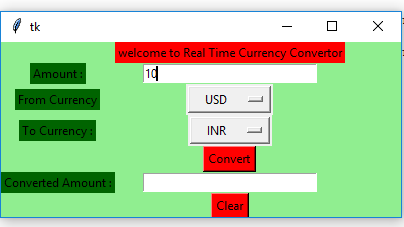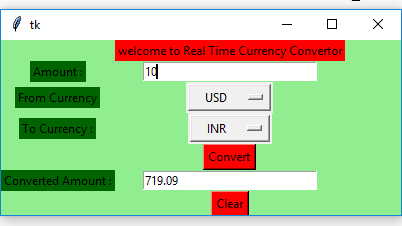Related Articles
Python | Real time currency convertor using Tkinter
• Difficulty Level : Medium
• Last Updated : 15 Sep, 2020

Prerequisites : Introduction to tkinter | Get the real time currency exchange rate

Python offers multiple options for developing GUI (Graphical User Interface). Out of all the GUI methods, tkinter is the most commonly used method. It is a standard Python interface to the Tk GUI toolkit shipped with Python. Python with tkinter outputs the fastest and easiest way to create the GUI applications.

To create a tkinter :

• Importing the module – tkinter
• Create the main window (container)
• Add any number of widgets to the main window.
• Apply the event Trigger on the widgets.

Let’s create a GUI based simple Real-time currency convertor (Using Alpha Vantage API) which can convert amounts from one currency to another currency.

Modules required:

```tkinter
requests
json
```

Below is the implementation :

 `# import all functions from the tkinter  ` `from` `tkinter ``import` `*` ` `  `# Create a GUI window ` `root ``=` `Tk() ` ` `  `# create a global variables  ` `variable1 ``=` `StringVar(root) ` `variable2 ``=` `StringVar(root) ` ` `  `# initialise the variables ` `variable1.``set``(``"currency"``) ` `variable2.``set``(``"currency"``) ` ` `  `     `  `# Function to perform real time conversion ` `# from one currency to another currency ` `def` `RealTimeCurrencyConversion(): ` ` `  `    ``# importing required libraries ` `    ``import` `requests, json ` ` `  `    ``# currency code ` `    ``from_currency ``=` `variable1.get() ` `    ``to_currency ``=` `variable2.get() ` ` `  `    ``# enter your api key here  ` `    ``api_key ``=` `"Your_Api_Key"` `     `  `    ``# base_url variable store base url  ` `    ``base_url ``=` `r``"https://www.alphavantage.co/query?function = CURRENCY_EXCHANGE_RATE"` ` `  `    ``# main_url variable store complete url ` `    ``main_url ``=` `base_url ``+` `"&from_currency ="` `+` `from_currency ``+`  `        ``"&to_currency ="` `+` `to_currency ``+` `"&apikey ="` `+` `api_key ` ` `  `    ``# get method of requests module  ` `    ``# return response object  ` `    ``req_ob ``=` `requests.get(main_url) ` ` `  `    ``# json method return json format ` `    ``# data into python dictionary data type. ` `     `  `    ``# result contains list of nested dictionaries ` `    ``result ``=` `req_ob.json() ` ` `  `    ``# parsing the required information ` `    ``Exchange_Rate ``=` `float``(result[``"Realtime Currency Exchange Rate"``] ` `                                              ``[``'5. Exchange Rate'``]) ` ` `  `    ``# get method of Entry widget ` `    ``# returns current text  as a ` `    ``# string from text entry box. ` `    ``amount ``=` `float``(Amount1_field.get()) ` ` `  `    ``# calculation for the conversion ` `    ``new_amount ``=` `round``(amount ``*` `Exchange_Rate, ``3``) ` ` `  `    ``# insert method inserting the  ` `    ``# value in the text entry box.  ` `    ``Amount2_field.insert(``0``, ``str``(new_amount)) ` ` `  ` `  `# Function for clearing the Entry field ` `def` `clear_all() : ` `    ``Amount1_field.delete(``0``, END)  ` `    ``Amount2_field.delete(``0``, END) ` `    `  ` `  `# Driver code ` `if` `__name__ ``=``=` `"__main__"` `: ` ` `  `    ``# Set the background colour of GUI window  ` `    ``root.configure(background ``=` `'light green'``)  ` `   `  `    ``# Set the configuration of GUI window (WidthxHeight) ` `    ``root.geometry(``"400x175"``)  ` `   `  `    ``# Create welcome to Real Time Currency Convertor label  ` `    ``headlabel ``=` `Label(root, text ``=` `'welcome to Real Time Currency Convertor'``,  ` `                      ``fg ``=` `'black'``, bg ``=` `"red"``)  ` ` `  `    ``# Create a "Amount :" label  ` `    ``label1 ``=` `Label(root, text ``=` `"Amount :"``, ` `                 ``fg ``=` `'black'``, bg ``=` `'dark green'``) ` `     `  `    ``# Create a "From Currency :" label  ` `    ``label2 ``=` `Label(root, text ``=` `"From Currency"``,  ` `                   ``fg ``=` `'black'``, bg ``=` `'dark green'``)  ` `   `  `    ``# Create a "To Currency: " label  ` `    ``label3 ``=` `Label(root, text ``=` `"To Currency :"``,  ` `                   ``fg ``=` `'black'``, bg ``=` `'dark green'``) ` ` `  `    ``# Create a "Converted Amount :" label  ` `    ``label4 ``=` `Label(root, text ``=` `"Converted Amount :"``,  ` `                   ``fg ``=` `'black'``, bg ``=` `'dark green'``) ` ` `  `    ``# grid method is used for placing  ` `    ``# the widgets at respective positions  ` `    ``# in table like structure .   ` `    ``headlabel.grid(row ``=` `0``, column ``=` `1``)  ` `    ``label1.grid(row ``=` `1``, column ``=` `0``)  ` `    ``label2.grid(row ``=` `2``, column ``=` `0``) ` `    ``label3.grid(row ``=` `3``, column ``=` `0``) ` `    ``label4.grid(row ``=` `5``, column ``=` `0``) ` `     `  `    ``# Create a text entry box  ` `    ``# for filling or typing the information.  ` `    ``Amount1_field ``=` `Entry(root)  ` `    ``Amount2_field ``=` `Entry(root) ` `      `  `    ``# ipadx keyword argument set width of entry space.  ` `    ``Amount1_field.grid(row ``=` `1``, column ``=` `1``, ipadx ``=``"25"``)  ` `    ``Amount2_field.grid(row ``=` `5``, column ``=` `1``, ipadx ``=``"25"``) ` ` `  `    ``# list of currency codes ` `    ``CurrenyCode_list ``=` `[``"INR"``, ``"USD"``, ``"CAD"``, ``"CNY"``, ``"DKK"``, ``"EUR"``] ` ` `  `    ``# create a drop down menu using OptionMenu function ` `    ``# which takes window name, variable and choices as ` `    ``# an argument. use * befor the name of the list, ` `    ``# to unpack the values ` `    ``FromCurrency_option ``=` `OptionMenu(root, variable1, ``*``CurrenyCode_list) ` `    ``ToCurrency_option ``=` `OptionMenu(root, variable2, ``*``CurrenyCode_list) ` `     `  `    ``FromCurrency_option.grid(row ``=` `2``, column ``=` `1``, ipadx ``=` `10``) ` `    ``ToCurrency_option.grid(row ``=` `3``, column ``=` `1``, ipadx ``=` `10``) ` `     `  `    ``# Create a Convert Button and attached  ` `    ``# with RealTimeCurrencyExchangeRate function  ` `    ``button1 ``=` `Button(root, text ``=` `"Convert"``, bg ``=` `"red"``, fg ``=` `"black"``, ` `                                ``command ``=` `RealTimeCurrencyConversion) ` `     `  `    ``button1.grid(row ``=` `4``, column ``=` `1``) ` ` `  `    ``# Create a Clear Button and attached  ` `    ``# with delete function  ` `    ``button2 ``=` `Button(root, text ``=` `"Clear"``, bg ``=` `"red"``,  ` `                     ``fg ``=` `"black"``, command ``=` `clear_all) ` `    ``button2.grid(row ``=` `6``, column ``=` `1``) ` `   `  `    ``# Start the GUI  ` `    ``root.mainloop()  `

Output :Attention geek! Strengthen your foundations with the Python Programming Foundation Course and learn the basics.

To begin with, your interview preparations Enhance your Data Structures concepts with the Python DS Course.

My Personal Notes arrow_drop_up
Recommended Articles
Page :